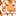# QlikView App Dev

Discussion Board for collaboration related to QlikView App Development.

Announcements
Now Live: Qlik Sense SaaS Simplified Authoring – Analytics Creation for Everyone: READ DETAILS
cancel
Showing results for
Did you mean:Creator

=count({<[Year of Expense] =  {"\$(vCurrentYear)"},

[Month of Expense]={'Jan'},

Keyplatopco = {"=sum([Expense Amount (USD)]*12) < 75000"},

[Project Activity Status] ={'Self-Perform','Active'}  >}  ID )

This logic was working until I added Month of Expense = Jan.

Keplatopco is calculated as below,

AutoNumber(RecordId&Year([Payment Date])) as Keyplatopco

do I need to add Month as well to Keyplatopco ?

AutoNumber(RecordId&Year([Payment Date])&Month([Payment Date])) as Keyplatopco

Any help is appreciated.

Thank you.

1 Solution

Accepted SolutionsMVP

Then adding month might make sense or try this

=count({<[Year of Expense] =  {"\$(vCurrentYear)"},

[Month of Expense]={'Jan'},

Keyplatopco = {"=sum({<[Month of Expense]={'Jan'}>}[Expense Amount (USD)]*12) < 75000"},

[Project Activity Status] ={'Self-Perform','Active'}  >}  ID )

7 RepliesChampion III

Can you try this?

=count({<[Year of Expense] =  {'\$(vCurrentYear)'},

[Month of Expense]={'Jan'},

Keyplatopco = {"=\$(= sum([Expense Amount (USD)]*12) < 75000)"},

[Project Activity Status] ={'Self-Perform','Active'}  >}  ID )MVP

Is this condition checked at the Month level?

=sum([Expense Amount (USD)]*12) < 75000MVP

I don't think dollar sign expansion is needed here... this is a search string which has to be between double quotesCreator
Author

ya...checking this condition at current year and then the Month level as for Month of Jan.MVP

Then adding month might make sense or try this

=count({<[Year of Expense] =  {"\$(vCurrentYear)"},

[Month of Expense]={'Jan'},

Keyplatopco = {"=sum({<[Month of Expense]={'Jan'}>}[Expense Amount (USD)]*12) < 75000"},

[Project Activity Status] ={'Self-Perform','Active'}  >}  ID )Master III

what is the format of the value in [Month of Expense]

?Creator
Author

Thank you as always